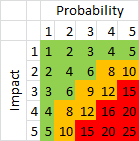# A simple method for estimating and evaluating risk

A simple way to calculate, evaluate and manage an identified risk is to look at two specific properties and that is its probability and impact.

## Calculating the risk

Let’s have a look at the steps in calculating the risk!

### Step 1 – Risk identification

The first step is to identify all risks. Simply collect all risks in a list with a short description.

E.g. ”Missing required resources” or ”Earthquake occurs”

### Step 2 – Probability and impact estimation

The next step is to estimate

1. the probability that the risk actually occurs and
2. the impact of the risk if it occurs.

The probability is a value between 1 (low) and 5 (high) stating the probability that the identified risk will occur.

The impact is a value between 1 (low) and 5 (high) indicating the impact of the identified risk if it occurs.

### Step 3 – Calculating the risk estimate

The final step is to calculate the total risk estimate for each risk.

Do this by multiplying the estimated impact with the estimated probability. The result should be a value between 1 and 25.

## Evaluating the risk estimate

Once you have calculated the risk estimate you need a way to evaluate the result.

One way to do this is to categorise them as indicated by the table below.

• Green – an estimate between 1 and 6 – acceptable risk level – keep a eye on these risks, plan and take actions to avoid them when possible
• Yellow – an estimate between 8 and 12 – tolerable risk level – plan and take actions avoid this risk
• Red – an estimate between 15 and 25 – intolerable risk level – take actions directly to avoid this riskRisk evaluation matrix

Once you have evaluated the risks you should track and make sure that the risks are handled in the correct order, i.e. highest risk(s) first.

How do you handle your risks? What method are you using? Leave a comment!